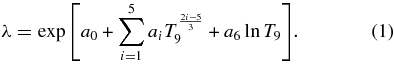# REACLIB Help

Main Page > REACLIB File Format

## REACLIB File Format

### REACLIB 1 Format

The REACLIB 1 (R1) file format is the default output of the REACLIB Database. Every three lines in the R1 file comprises of an entry can be of two different types. It is either a header entry or a set entry. Every line in a REACLIB file is 74 characters long and is padding with spaces. Because of this a blank line is actually 74 space characters.

A header entry describes which chapter the rate is in. A chapter is an integer number that describes the equation that is used to tell how many nuclides are involved in the reaction and which are reactants and products (Table 1). The header entry starts with a line containing the chapter number and the next two lines are blank.

Historically, chapters 9, 10, and 11 didn't exist and chapter 8 handled an additional reaction type. Chapter 8 handled the rates that were either e1 + e2 + e3 → e4 or e1 + e2 + e3 → e4 + e5. In order to handle reverse rates properly Chapter 8 has been split into chapters 8 and 9 and chapter 10 was added. Chapter 11 has been added to support rates of the type e1 → e2 + e3 + e4 + e5. One can download either the historical REACLIB without chapters 9-11, or the modern REACLIB with chapters 9-11.

Chapter Equation
1 e1 → e2
2 e1 → e2 + e3
3 e1 → e2 + e3 + e4
4 e1 + e2 → e3
5 e1 + e2 → e3 + e4
6 e1 + e2 → e3 + e4 + e5
7 e1 + e2 → e3 + e4 + e5 + e6
8 e1 + e2 + e3 → e4
9 e1 + e2 + e3 → e4 + e5
10 e1 + e2 + e3 + e4 → e5 + e6
11 e1 → e2 + e3 + e4 + e5
Table 1: Reaclib Chapters

#### Set

The set entry describes one set for a specific reaction. One or more sets are used to define a rate. In the cases there are multiple sets the sets will be sequential entries in the REACLIB file. Each rate is defined as:where: T9 is the temperature in Gigakelvin and ai are the entries in the set.

The first line of the set entry is as follows:

• Five(5) space characters.
• Six(6) fields of five(5) characters that contain the nuclides for the rate padded with spaces.
• Eight(8) spaces.
• Four(4) characters that contains the set label.
• A one(1) character flag denoting:
• blank or n: A non-resonant rate.
• r: A resonant rate.
• w: A weak rate.
• A one(1) character flag that is set to 'v' when this is a reverse rate.
• The "v" flag shows reverse rates in which detailed balance (without partition functions) was used to derive the reaction rate. Therefore, rates with this flag must be corrected to include partition function modifications.
• Three(3) space characters.
• A twelve(12) character field that contains the Q value for the reaction. 0.00000e+00.
• Ten(10) space characters.

The second line of the set entry contains the first four ai (a0, a1, a2, a3) values in that set. Each of these fields are 13 characters (0.000000e+00). Followed by 22 space characters.

The third line of the set entry contains the last three ai (a4, a5, a6) values in that set. Each of these fields are 13 characters (0.000000e+00). Followed by 35 space characters.

format(i1,4x,6a5,8x,a4,a1,a1,3x,1pe12.5)
format(4e13.6)
format(3e13.6)

### REACLIB 2 Format

The REACLIB 2 (R2) format is exactly like R1 but the header entry (chapter #) occurs before every set entry.

If you have any reaclib/database questions or problems please email us. Send Email
Last updated by cyburt on November 29th 2010 at 09:39